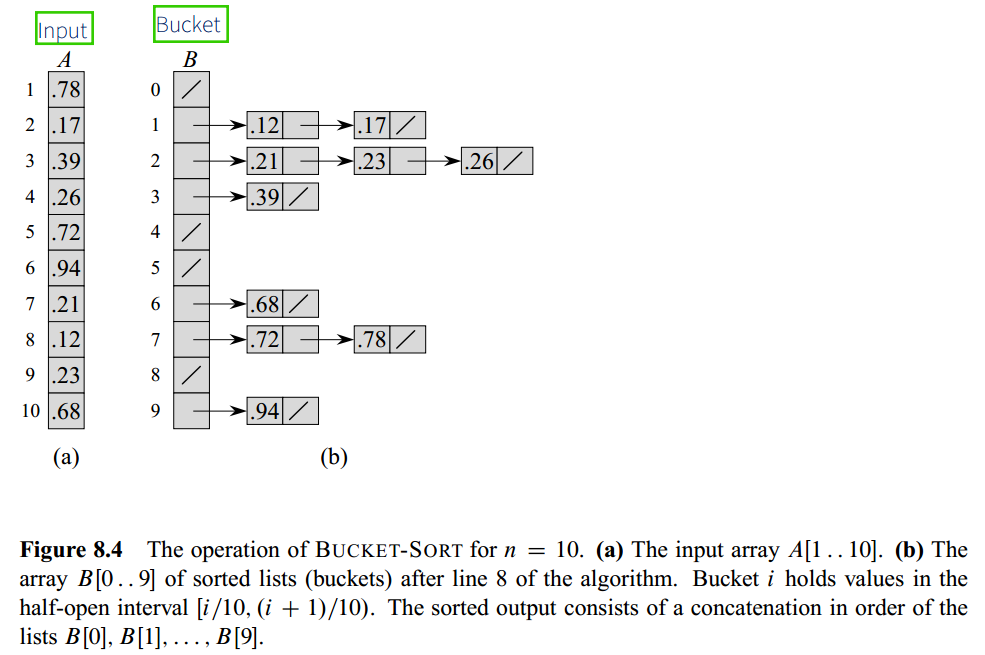# Bucket Sort

Updated:

⚠️ This post was created when I was in high school and is no longer maintained.

## 1. Intro

1. Bucket sort assumes that the input is drawn from a uniform distribution and has an average-case running time of O(n). ( Unform distribution )
2. Counting sort assumes that the input consists of integers in a small range, bucket sort assumes that the input is generated by a random process that distributes elements uniformly and independently over the interval [0, 1)
3. Bucket: This algorithm divides the interval [0, 1) into n equal-sized subintervals.
4. We sort the numbers in each bucket and then go through the buckets in order, listing the elements in each## 2. Pseudocode

algorithm BucketSort(A):
n = A.length
Let B[0...(n-1)] be a new array

For i = 0 to (n-1) do
Make B[i] an empty list
For i = 1 to n do
Insert A[i] into B[floor(n * A[i])]
For i = 0 to (n-1) do
InsertionSort(B[i], B[i].length)

Concatenate the lists B, B..., B[n-1] togther in order


## 3. Time Complexity

1. Running time: $T(n) = \Theta(n)+\sum^{n-1}_{i=0}O(n_i^2)$
2. Average case: $\Theta(n)+n\cdot O(2-1/n) =\Theta(n)$

## 4. Code

import scala.collection.mutable.ArrayBuffer
import scala.math._

object BucketSortAlgo {

def insertionSort(array: Array[Double], n: Int) : Array[Double] = {
for (j <- 1 until n) {
var key = array(j)
var i = j - 1
while (i >= 0 && array(i) > key) {
array(i+1) = array(i)
i -= 1
}
array(i+1) = key
}
return array
}

def bucketSort(array: Array[Double]): ArrayBuffer[Double] = {
val bucket_num = array.length
val bucket = ArrayBuffer.fill[Double](bucket_num, 0)(0)
val result = ArrayBuffer[Double]()

var i = 0
for (i <- 0 until bucket_num) {
bucket(floor(array(i)).toInt) += array(i)
}
for (i <- 0 until bucket_num) {
bucket(i) = insertionSort(bucket(i).to[Array], bucket(i).length).to[ArrayBuffer]
}
for (i <- 0 until bucket_num) {
result ++= bucket(i)
}
return result
}

def main(args: Array[String]): Unit = {
var array = Array(0.25, 0.49, 0.667, 0.41, 0.988, 0.456, 0.245, 0.565, 0.8277, 0.351, 0.12, 0.52, 0.51, 0, 0.93, 0.77)
println("\nBefore sorting:")
println(array.mkString(" "))
println("\nAfter sorting:")
println(bucketSort(array).mkString(" "))
}
}

# Python
import math

def insertion_sort(array, n):
for j in range(1, n):
key = array[j]
i = j - 1
while i >= 0 and array[i] > key:
array[i], array[i+1] = array[i+1], array[i]
i -= 1
array[i+1] = key

def bucket_sort(array):
ans = []
n = len(array)
bucket = [i[:] for i in [[]] * n]

for i in range(n):
bucket[math.floor(array[i] * n)].append(array[i])
for j in range(n):
insertion_sort(bucket[j], len(bucket[j]))
for i in range(n):
ans.extend(bucket[i])
print(ans)

if __name__ == "__main__":
array = [0.25, 0.49, 0.667, 0.41, 0.988, 0.456, 0.245, 0.565, 0.8277, 0.351, 0.12, 0.52, 0.51, 0, 0.93, 0.77]
print("\nBefore sorting:", array)

print("\nAfter sorting:", bucket_sort(array))


Tags: